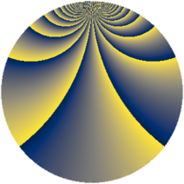# Properties

 Label 240.2.bbLevel $240$ Weight $2$ Character orbit 240.bb Rep. character $\chi_{240}(173,\cdot)$ Character field $\Q(\zeta_{4})$ Dimension $88$ Newform subspaces $1$ Sturm bound $96$ Trace bound $0$

# Related objects

## Defining parameters

 Level: $$N$$ $$=$$ $$240 = 2^{4} \cdot 3 \cdot 5$$ Weight: $$k$$ $$=$$ $$2$$ Character orbit: $$[\chi]$$ $$=$$ 240.bb (of order $$4$$ and degree $$2$$) Character conductor: $$\operatorname{cond}(\chi)$$ $$=$$ $$240$$ Character field: $$\Q(i)$$ Newform subspaces: $$1$$ Sturm bound: $$96$$ Trace bound: $$0$$

## Dimensions

The following table gives the dimensions of various subspaces of $$M_{2}(240, [\chi])$$.

Total New Old
Modular forms 104 104 0
Cusp forms 88 88 0
Eisenstein series 16 16 0

## Trace form

 $$88q - 4q^{4} - 4q^{6} + O(q^{10})$$ $$88q - 4q^{4} - 4q^{6} - 12q^{10} + 4q^{12} - 4q^{15} - 12q^{16} + 16q^{18} - 8q^{19} - 4q^{21} - 20q^{22} - 12q^{24} - 36q^{28} - 16q^{30} - 16q^{31} - 4q^{33} + 28q^{34} - 20q^{36} + 24q^{39} - 4q^{40} - 20q^{42} - 40q^{43} + 8q^{45} - 36q^{46} + 16q^{48} - 4q^{51} + 24q^{52} - 24q^{54} + 12q^{57} + 44q^{58} - 56q^{60} - 24q^{61} - 32q^{63} - 28q^{64} + 12q^{66} - 8q^{67} - 12q^{69} - 28q^{70} + 64q^{72} - 24q^{75} - 36q^{76} + 20q^{78} - 8q^{81} - 48q^{82} + 48q^{84} - 24q^{85} - 12q^{87} + 60q^{88} + 76q^{90} - 8q^{91} - 20q^{94} + 48q^{96} - 8q^{97} - 32q^{99} + O(q^{100})$$

## Decomposition of $$S_{2}^{\mathrm{new}}(240, [\chi])$$ into newform subspaces

Label Dim. $$A$$ Field CM Traces $q$-expansion
$$a_2$$ $$a_3$$ $$a_5$$ $$a_7$$
240.2.bb.a $$88$$ $$1.916$$ None $$0$$ $$0$$ $$0$$ $$0$$